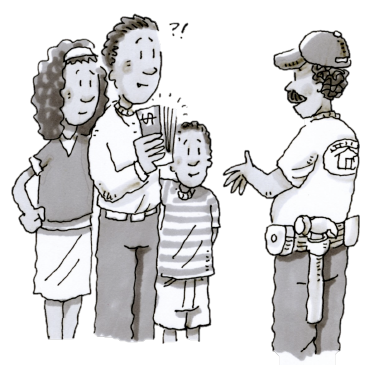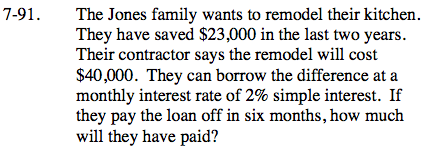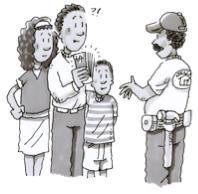Home > CC2MN > Chapter cc27 > Lesson cc27.1.8 > Problem7-91

7-91.The Jones family wants to remodel their kitchen. They have saved $23,000 in the last two years. Their contractor says the remodel will cost$40,000. They can borrow the difference at a monthly interest rate of 2% simple interest. If they pay the loan off in six months, how much will they have paid? Homework Help ✎First calculate how much money the Jones need to borrow.
$40,000 −$23,000 = $17,000 Calculate the amount of interest that is added per month.$17,000(0.02) = $340 Since the Jones can pay off the loan in 6 months, multiply the amount of interest added per month by 6.$340(6) = $2,040 Finally, add that amount to the original to find the amount of money the Jones need to pay.$17,000 + $2,040 =$19,040

Do you see how the simple interest formula was used?
I = (17,000)(0.02)(6)